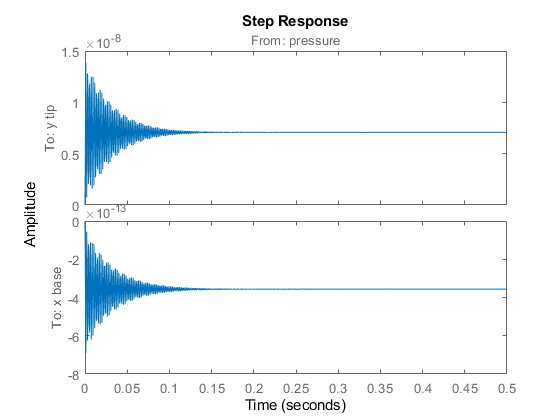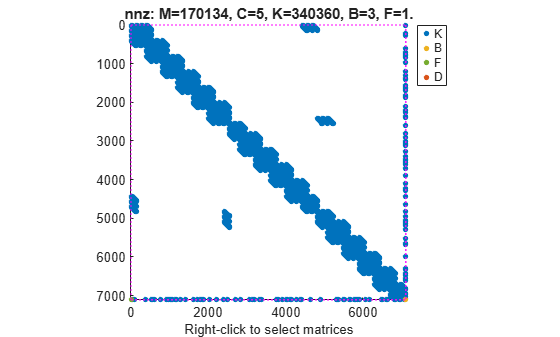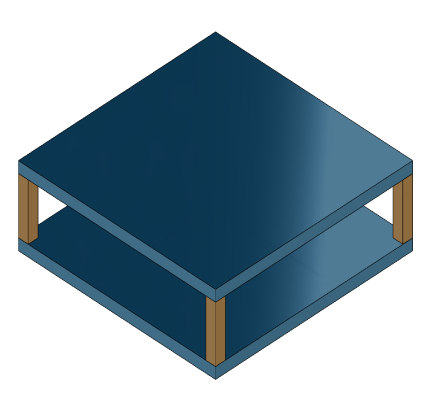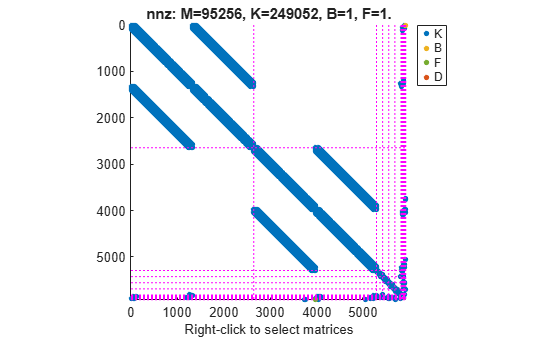# mechss

Sparse second-order state-space model

## Description

Use `mechss` to represent second-order sparse models using matrices obtained from your finite element analysis (FEA) package. Such sparse models arise from finite element analysis (FEA) and are useful in fields like structural analysis, fluid flow, heat transfer and electromagnetics. The resultant matrices from this type of modeling are quite large with a sparse pattern. Hence, using `mechss` is an efficient way to represent such large sparse state-space models in MATLAB® to perform linear analysis. You can also use `mechss` to convert a first-order `sparss` model object or other dynamic system models to a `mechss` object.

You can use `mechss` model objects to represent SISO or MIMO state-space models in continuous time or discrete time. In continuous time, a second-order sparse mass-spring-damper model is represented in the following form:

Here, the full state vector is given by $\left[q,\stackrel{˙}{q}\right]$ where $q$ and $\stackrel{˙}{q}$ are the displacement and velocity vectors. `u` and `y` represent the inputs and outputs, respectively. `M`, `C` and `K` represent the mass, damping and stiffness matrices, respectively. `B` is the input matrix while `F` and `G` are the output matrices for displacement and velocity, respectively. `D` is the input-to-output matrix.

You can use a `mechss` object to:

• Perform time-domain and frequency-domain response analysis.

• Specify signal-based connections with other LTI models.

• Specify physical interfaces between components using the `interface` command.

## Creation

### Syntax

``sys = mechss(M,C,K,B,F,G,D)``
``sys = mechss(M,C,K,B,F,G,D,ts)``
``sys = mechss(M,C,K)``
``sys = mechss(D)``
``sys = mechss(___,Name,Value)``
``sys = mechss(ltiSys)``

### Description

example

````sys = mechss(M,C,K,B,F,G,D)` creates a `mechss` object representing this continuous-time second-order mass-spring-damper model: Here, `M`, `C`, and `K` represent the mass, damping, and stiffness matrices, respectively. `B` is the input-to-state matrix while `F` and `G` are the displacement-to-output and velocity-to-output matrices resulting from the two components of the state `x`. `D` is the input-to-output matrix. You can set `M` to `[]` when the mass matrix is an identity matrix. Set `G` and `D` to `[]` or omit them when they are empty.```

example

````sys = mechss(M,C,K,B,F,G,D,ts)` uses the sample time `ts` to create a `mechss` object representing this discrete-time second-order mass-spring-damper model: To leave the sample time unspecified, set `ts` to `-1`.```

example

````sys = mechss(M,C,K)` creates a `mechss` model object with the following assumptions: Identity matrices for `B` and `F` with the same size as mass matrix `M`Matrices of zeros for `G` and `D` ```

example

````sys = mechss(D)` creates a `mechss` model object that represents the static gain `D`. The output sparse state-space model is equivalent to `mechss([],[],[],[],[],[],D)`.```

example

````sys = mechss(___,Name,Value)` sets properties of the second-order sparse state-space model using one or more name-value pair arguments. Use this syntax with any of the previous input-argument combinations.```

example

````sys = mechss(ltiSys)` converts the dynamic system model `ltiSys` to a second-order sparse state-space model.```

### Input Arguments

expand all

Mass matrix, specified as an `Nq`-by-`Nq` sparse matrix, where `Nq` is the number of nodes. This input sets the value of property M.

Damping matrix, specified as an `Nq`-by-`Nq` sparse matrix, where `Nq` is the number of nodes. You can also set `C=[]` to specify zero damping. This input sets the value of property C.

Stiffness matrix, specified as an `Nq`-by-`Nq` sparse matrix, where `Nq` is the number of nodes. This input sets the value of property K.

Input-to-state matrix, specified as an `Nq`-by-`Nu` sparse matrix, where `Nq` is the number of nodes and `Nu` is the number of inputs. This input sets the value of property B.

Displacement-to-output matrix, specified as an `Ny`-by-`Nq` sparse matrix, where `Nq` is the number of nodes and `Ny` is the number of outputs. This input sets the value of property F.

Velocity-to-output matrix, specified as an `Ny`-by-`Nq` sparse matrix, where `Nq` is the number of nodes and `Ny` is the number of outputs. This input sets the value of property G.

Input-to-output matrix, specified as an `Ny`-by-`Nu` sparse matrix, where `Ny` is the number of outputs and `Nu` is the number of inputs. This input sets the value of property D.

Sample time, specified as a scalar. For more information see the Ts property.

Dynamic system to convert to second-order sparse state-space form, specified as a SISO or MIMO dynamic system model or array of dynamic system models. Dynamic systems that you can convert include:

### Output Arguments

expand all

Output system model, returned as a `mechss` model object.

## Properties

expand all

Mass matrix, specified as an `Nq`-by-`Nq` sparse matrix where, `Nq` is the number of nodes.

Damping matrix, specified as an `Nq`-by-`Nq` sparse matrix where, `Nq` is the number of nodes.

Damping matrix, specified as an `Nq`-by-`Nq` sparse matrix where, `Nq` is the number of nodes.

Input-to-state matrix, specified as an `Nq`-by-`Nu` sparse matrix where, `Nq` is the number of nodes and `Nu` is the number of inputs.

Displacement-to-output matrix, specified as an `Ny`-by-`Nq` sparse matrix where, `Nq` is the number of nodes and `Ny` is the number of outputs.

Velocity-to-output matrix, specified as an `Ny`-by-`Nq` sparse matrix where, `Nq` is the number of nodes and `Ny` is the number of outputs.

Input-to-output matrix, specified as an `Ny`-by-`Nu` sparse matrix where, `Ny` is the number of outputs and `Nu` is the number of inputs. `D` is also called the static gain matrix, and represents the ratio of the output to the input in steady state condition.

State partition information containing state vector components, interfaces between components and internal signal connecting components, specified as a structure array with the following fields:

• `Type` — Type includes component, signal or physical interface

• `Name` — Name of the component, signal or physical interface

• `Size` — Number of states or nodes in the partition

You can view the partition information of the sparse state-space model using `showStateInfo`. You can also sort and order the partitions in your sparse model using `xsort`.

Options for model analysis, specified as a structure with the following fields:

• `UseParallel` — Set this option to `true` to enable parallel computing and `false` to disable it. Parallel computing is disabled by default. The `UseParallel` option requires a Parallel Computing Toolbox™ license.

• `DAESolver` — Use this option to select the type of differential algebraic equation (DAE) solver. The following DAE solvers are available:

• `'trbdf2'` — Fixed-step solver with an accuracy of `o(h^2)`, where `h` is the step size. This is the default solver for the `mechss` model object.

• `'trbdf3'` — Fixed-step solver with an accuracy of `o(h^3)`, where `h` is the step size.

• `'hht'` — Fixed-step solver with an accuracy of `o(h^2)`, where `h` is the step size.

Reducing the step size increases accuracy and extends the frequency range where numerical damping is negligible. `'hht'` is the fastest but can run into difficulties with high initial acceleration (for example, an impulse response with initial jerk). `'trbdf2'` requires about twice as many computations as `'hht'` and `'trbdf3'` requires another 50% more computations than `'trbdf2'`.

For an example, see Time and Frequency Response of Sparse Second-Order Model.

Internal delays in the model, specified as a vector. Internal delays arise, for example, when closing feedback loops on systems with delays, or when connecting delayed systems in series or parallel. For more information about internal delays, see Closing Feedback Loops with Time Delays.

For continuous-time models, internal delays are expressed in the time unit specified by the `TimeUnit` property of the model. For discrete-time models, internal delays are expressed as integer multiples of the sample time `Ts`. For example, `InternalDelay = 3` means a delay of three sampling periods.

You can modify the values of internal delays using the property `InternalDelay`. However, the number of entries in `sys.InternalDelay` cannot change, because it is a structural property of the model.

Input delay for each input channel, specified as one of the following:

• Scalar — Specify the input delay for a SISO system or the same delay for all inputs of a multi-input system.

• `Nu`-by-1 vector — Specify separate input delays for input of a multi-input system, where `Nu` is the number of inputs.

For continuous-time systems, specify input delays in the time unit specified by the `TimeUnit` property. For discrete-time systems, specify input delays in integer multiples of the sample time, `Ts`.

Output delay for each output channel, specified as one of the following:

• Scalar — Specify the output delay for a SISO system or the same delay for all outputs of a multi-output system.

• `Ny`-by-1 vector — Specify separate output delays for output of a multi-output system, where `Ny` is the number of outputs.

For continuous-time systems, specify output delays in the time unit specified by the `TimeUnit` property. For discrete-time systems, specify output delays in integer multiples of the sample time, `Ts`.

Sample time, specified as:

• `0` for continuous-time systems.

• A positive scalar representing the sampling period of a discrete-time system. Specify `Ts` in the time unit specified by the `TimeUnit` property.

• `-1` for a discrete-time system with an unspecified sample time.

Note

Changing `Ts` does not discretize or resample the model. To convert between continuous-time and discrete-time representations, use `c2d` and `d2c`. To change the sample time of a discrete-time system, use `d2d`.

Time variable units, specified as one of the following:

• `'nanoseconds'`

• `'microseconds'`

• `'milliseconds'`

• `'seconds'`

• `'minutes'`

• `'hours'`

• `'days'`

• `'weeks'`

• `'months'`

• `'years'`

Changing `TimeUnit` has no effect on other properties, but changes the overall system behavior. Use `chgTimeUnit` to convert between time units without modifying system behavior.

Input channel names, specified as one of the following:

• A character vector, for single-input models.

• A cell array of character vectors, for multi-input models.

• `''`, no names specified for any input channels.

Alternatively, you can assign input names for multi-input models using automatic vector expansion. For example, if `sys` is a two-input model, enter:

`sys.InputName = 'controls';`

The input names automatically expand to `{'controls(1)';'controls(2)'}`.

You can use the shorthand notation `u` to refer to the `InputName` property. For example, `sys.u` is equivalent to `sys.InputName`.

Use `InputName` to:

• Identify channels on model display and plots.

• Extract subsystems of MIMO systems.

• Specify connection points when interconnecting models.

Input channel units, specified as one of the following:

• A character vector, for single-input models.

• A cell array of character vectors, for multi-input models.

• `''`, no units specified for any input channels.

Use `InputUnit` to specify input signal units. `InputUnit` has no effect on system behavior.

Input channel groups, specified as a structure. Use `InputGroup` to assign the input channels of MIMO systems into groups and refer to each group by name. The field names of `InputGroup` are the group names and the field values are the input channels of each group. For example:

```sys.InputGroup.controls = [1 2]; sys.InputGroup.noise = [3 5];```

creates input groups named `controls` and `noise` that include input channels `1` and `2`, and `3` and `5`, respectively. You can then extract the subsystem from the `controls` inputs to all outputs using:

`sys(:,'controls')`

By default, `InputGroup` is a structure with no fields.

Output channel names, specified as one of the following:

• A character vector, for single-output models.

• A cell array of character vectors, for multi-output models.

• `''`, no names specified for any output channels.

Alternatively, you can assign output names for multi-output models using automatic vector expansion. For example, if `sys` is a two-output model, enter:

`sys.OutputName = 'measurements';`

The output names automatically expand to `{'measurements(1)';'measurements(2)'}`.

You can also use the shorthand notation `y` to refer to the `OutputName` property. For example, `sys.y` is equivalent to `sys.OutputName`.

Use `OutputName` to:

• Identify channels on model display and plots.

• Extract subsystems of MIMO systems.

• Specify connection points when interconnecting models.

Output channel units, specified as one of the following:

• A character vector, for single-output models.

• A cell array of character vectors, for multi-output models.

• `''`, no units specified for any output channels.

Use `OutputUnit` to specify output signal units. `OutputUnit` has no effect on system behavior.

Output channel groups, specified as a structure. Use `OutputGroup`to assign the output channels of MIMO systems into groups and refer to each group by name. The field names of `OutputGroup` are the group names and the field values are the output channels of each group. For example:

```sys.OutputGroup.temperature = ; sys.InputGroup.measurement = [3 5];```

creates output groups named `temperature` and `measurement` that include output channels `1`, and `3` and `5`, respectively. You can then extract the subsystem from all inputs to the `measurement` outputs using:

`sys('measurement',:)`

By default, `OutputGroup` is a structure with no fields.

User-specified text that you want to associate with the system, specified as a character vector or cell array of character vectors. For example, `'System is MIMO'`.

User-specified data that you want to associate with the system, specified as any MATLAB data type.

System name, specified as a character vector. For example, `'system_1'`.

Sampling grid for model arrays, specified as a structure array.

Use `SamplingGrid` to track the variable values associated with each model in a model array.

Set the field names of the structure to the names of the sampling variables. Set the field values to the sampled variable values associated with each model in the array. All sampling variables must be numeric scalars, and all arrays of sampled values must match the dimensions of the model array.

For example, you can create an 11-by-1 array of linear models, `sysarr`, by taking snapshots of a linear time-varying system at times `t = 0:10`. The following code stores the time samples with the linear models.

` sysarr.SamplingGrid = struct('time',0:10)`

Similarly, you can create a 6-by-9 model array, `M`, by independently sampling two variables, `zeta` and `w`. The following code maps the `(zeta,w)` values to `M`.

```[zeta,w] = ndgrid(<6 values of zeta>,<9 values of w>) M.SamplingGrid = struct('zeta',zeta,'w',w)```

By default, `SamplingGrid` is a structure with no fields.

## Object Functions

The following lists show functions you can use with `mechss` model objects.

expand all

 `sparss` Sparse first-order state-space model `getx0` Map initial conditions from a `mechss` object to a `sparss` object `full` Convert sparse models to dense storage `imp2exp` Convert implicit linear relationship to explicit input-output relation `inv` Invert models `getDelayModel` State-space representation of internal delays
 `sparssdata` Access first-order sparse state-space model data `mechssdata` Access second-order sparse state-space model data `showStateInfo` State partition information `spy` Visualize sparsity pattern of a `sparss` model object
 `step` Step response plot of dynamic system; step response data `impulse` Impulse response plot of dynamic system; impulse response data `initial` Initial condition response of state-space model `lsim` Plot simulated time response of dynamic system to arbitrary inputs; simulated response data `bode` Bode plot of frequency response, or magnitude and phase data `nyquist` Nyquist plot of frequency response `nichols` Nichols chart of frequency response `sigma` Singular values plot of dynamic system `passiveplot` Compute or plot passivity index as function of frequency `dcgain` Low-frequency (DC) gain of LTI system `evalfr` Evaluate frequency response at given frequency `freqresp` Frequency response over grid
 `interface` Specify physical connections between components of `mechss` model `xsort` Sort states based on state partition `feedback` Feedback connection of multiple models `parallel` Parallel connection of two models `append` Group models by appending their inputs and outputs `connect` Block diagram interconnections of dynamic systems `lft` Generalized feedback interconnection of two models (Redheffer star product) `series` Series connection of two models

## Examples

collapse all

For this example, consider the sparse matrices for the 3-D beam model subjected to an impulsive point load at its tip in the file `sparseBeam.mat`.

Extract the sparse matrices from `sparseBeam.mat`.

`load('sparseBeam.mat','M','K','B','F','G','D');`

Create the `mechss` model object by specifying `[]` for matrix `C`, since there is no damping.

`sys = mechss(M,[],K,B,F,G,D)`
```Sparse continuous-time second-order model with 3 outputs, 1 inputs, and 3408 nodes. Use "spy" and "showStateInfo" to inspect model structure. Type "properties('mechss')" for a list of model properties. Type "help mechssOptions" for available solver options for this model. ```

The output `sys` is a `mechss` model object containing a 3-by-1 array of sparse models with 3408 nodes, 1 input, and 3 outputs.

You can use the `spy` command to visualize the sparsity of the `mechss` model object.

`spy(sys)`For this example, consider the sparse matrices of the discrete system in the file `discreteSOSparse.mat`.

Load the sparse matrices from `discreteSOSparse.mat`.

`load('discreteSOSparse.mat','M','C','K','B','F','G','D','ts');`

Create the discrete-time `mechss` model object by specifying the sample time `ts`.

`sys = mechss(M,C,K,B,F,G,D,ts)`
```Sparse discrete-time second-order model with 1 outputs, 1 inputs, and 28408 nodes. Use "spy" and "showStateInfo" to inspect model structure. Type "properties('mechss')" for a list of model properties. Type "help mechssOptions" for available solver options for this model. ```

The output `sys` is a discrete-time `mechss` model object with 28408 nodes, 1 input, and 1 output.

You can use the `spy` command to visualize the sparsity pattern of the `mechss` model object. You can right-click on the plot to select matrices to be displayed.

`spy(sys)`For this example, consider `sparseSOArray.mat` which contains three sets of sparse matrices that define multiple sparse second-order state-space models.

Extract the data from `sparseSOArray.mat`.

`load('sparseSOArray.mat');`

Preallocate a 3-by-1 array of `mechss` models.

`sys = mechss(zeros(1,1,3));`

Next, use indexed assignment to populate the 3-by-1 array with sparse second-order models.

```sys(:,:,1) = mechss(M1,[],K1,B1,F1,G1,[]); sys(:,:,2) = mechss(M2,[],K2,B2,F2,G2,[]); sys(:,:,3) = mechss(M3,[],K3,B3,F3,G3,[]); size(sys)```
```3x1 array of sparse second-order models. Each model has 1 outputs, 1 inputs, and between 385 and 738 nodes. ```

Alternatively, you can also create an array of sparse second-order models using the `stack` command when you have models with the same I/O sizes.

Create a static gain MIMO sparse second-order state-space model.

Consider the following two-input, three-output static gain matrix:

`$D=\left[\begin{array}{cc}1& 5\\ 2& 3\\ 5& 9\end{array}\right]$`

Specify the gain matrix and create the static gain sparse second-order state-space model.

```D = [1,5;2,3;5,9]; sys = mechss(D); size(sys)```
```Sparse second-order model with 3 outputs, 2 inputs, and 0 nodes. ```

For this example, consider `sparseSOSignal.mat` which contains the mass, stiffness, and damping sparse matrices.

Load the sparse matrices from `sparseSOSignal.mat` and create the sparse second-order model object.

```load('sparseSOModel.mat','M','C','K'); sys = mechss(M,C,K);```

`mechss` creates the model object `sys` with the following assumptions:

• Identity matrices for `B` and `F` with the same size as mass matrix `M`.

• Zero matrices for `G` and `D`.

For this example, consider `sparssModel.mat` that contains a `sparss` model object `ltiSys`.

Load the `sparss` model object from `sparssModel.mat`.

```load('sparssModel.mat','ltiSys'); ltiSys```
```Sparse continuous-time state-space model with 1 outputs, 1 inputs, and 354 states. Use "spy" and "showStateInfo" to inspect model structure. Type "properties('sparss')" for a list of model properties. Type "help sparssOptions" for available solver options for this model. ```

Use the `mechss` command to convert to `mechss` model object representation.

`sys = mechss(ltiSys)`
```Sparse continuous-time second-order model with 1 outputs, 1 inputs, and 354 nodes. Use "spy" and "showStateInfo" to inspect model structure. Type "properties('mechss')" for a list of model properties. Type "help mechssOptions" for available solver options for this model. ```

For this example, consider `tuningForkData.mat` that contains the sparse second-order model of a tuning fork being struck gently but quickly on one of its tines. The system has one input, the pressure applied on one of its tines, which results in two outputs - the displacements at the tip and base of the tuning fork.

Load the sparse matrices from `tuningForkData.mat` into the workspace and create the `mechss` model object.

```load('tuningForkData.mat','M','K','B','F'); sys = mechss(M,[],K,B,F,'InputName','pressure','Outputname',{'y tip','x base'})```

Next, set solver options for the model by setting the `UseParallel` parameter to `true` and the `DAESolver` to use `trbdf3`. Use `spy` to inspect the model structure. Enabling parallel computing requires a Parallel Computing Toolbox™ license.

```sys.SolverOptions.UseParallel = true; sys.SolverOptions.DAESolver = 'trbdf3'; spy(sys)```You can also use `showStateInfo` to examine the components.

`showStateInfo(sys)`

Use `step` to obtain the step response plot of the system. You need to provide the time vector or final time for sparse models.

```t = linspace(0,0.5,1000); step(sys,t)```Next, obtain the Bode plot to examine the frequency response. You need to provide the frequency vector for sparse models.

```w = logspace(1,5,1000); bode(sys,w), grid```For this example, consider `sparseSOSignal.mat` that contains a sparse second-order model. Define an actuator, sensor, and controller and connect them together with the plant in a feedback loop.

Load the sparse matrices and create the `mechss` object.

```load sparseSOSignal.mat plant = mechss(M,C,K,B,F,[],[],'Name','Plant');```

Next, create an actuator and sensor using transfer functions.

```act = tf(1,[1 0.5 3],'Name','Actuator'); sen = tf(1,[0.02 7],'Name','Sensor');```

Create a PID controller object for the plant.

`con = pid(1,1,0.1,0.01,'Name','Controller');`

Use the `feedback` command to connect the plant, sensor, actuator, and controller in a feedback loop.

`sys = feedback(sen*plant*act*con,1)`
```Sparse continuous-time second-order model with 1 outputs, 1 inputs, and 7111 nodes. Use "spy" and "showStateInfo" to inspect model structure. Type "properties('mechss')" for a list of model properties. Type "help mechssOptions" for available solver options for this model. ```

The resultant system `sys` is a `mechss` object since `mechss` objects take precedence over all other model object types.

Use `showStateInfo` to view the component and signal groups.

`showStateInfo(sys)`
```The state groups are: Type Name Size ------------------------------- Component Sensor 1 Component Plant 7102 Signal 1 Component Actuator 2 Signal 1 Component Controller 2 Signal 1 Signal 1 ```

Use `xsort` to sort the components and signals, and then view the component and signal groups.

```sysSort = xsort(sys); showStateInfo(sysSort)```
```The state groups are: Type Name Size ------------------------------- Component Sensor 1 Component Plant 7102 Component Actuator 2 Component Controller 2 Signal 4 ```

Observe that the components are now ordered before the signal partition. The signals are now sorted and grouped together in a single partition.

You can also visualize the sparsity pattern of the resultant system using `spy`.

`spy(sysSort)`For this example, consider a structural model that consists of two square plates connected with pillars at each vertex as depicted in the figure below. The lower plate is attached rigidly to the ground while the pillars are attached rigidly to each vertex of the square plate.Load the finite element model matrices contained in `platePillarModel.mat` and create the sparse second-order model representing the above system.

```load('platePillarModel.mat') sys = ... mechss(M1,[],K1,B1,F1,'Name','Plate1') + ... mechss(M2,[],K2,B2,F2,'Name','Plate2') + ... mechss(Mp,[],Kp,Bp,Fp,'Name','Pillar3') + ... mechss(Mp,[],Kp,Bp,Fp,'Name','Pillar4') + ... mechss(Mp,[],Kp,Bp,Fp,'Name','Pillar5') + ... mechss(Mp,[],Kp,Bp,Fp,'Name','Pillar6');```

Use `showStateInfo` to examine the components of the `mechss` model object.

`showStateInfo(sys)`
```The state groups are: Type Name Size ---------------------------- Component Plate1 2646 Component Plate2 2646 Component Pillar3 132 Component Pillar4 132 Component Pillar5 132 Component Pillar6 132 ```

Now, load the interfaced node index data from `nodeData.mat` and use `interface` to create the physical connections between the two plates and the four pillars. `nodes` is a `6x7` cell array where the first two rows contain node index data for the first and second plates while the remaining four rows contain index data for the four pillars.

```load('nodeData.mat','nodes') for i=3:6 sys = interface(sys,"Plate1",nodes{1,i},"Pillar"+i,nodes{i,1}); sys = interface(sys,"Plate2",nodes{2,i},"Pillar"+i,nodes{i,2}); end```

Specify connection between the bottom plate and the ground.

`sysCon = interface(sys,"Plate2",nodes{2,7});`

Use `showStateInfo` to confirm the physical interfaces.

`showStateInfo(sysCon)`
```The state groups are: Type Name Size ----------------------------------- Component Plate1 2646 Component Plate2 2646 Component Pillar3 132 Component Pillar4 132 Component Pillar5 132 Component Pillar6 132 Interface Plate1-Pillar3 12 Interface Plate2-Pillar3 12 Interface Plate1-Pillar4 12 Interface Plate2-Pillar4 12 Interface Plate1-Pillar5 12 Interface Plate2-Pillar5 12 Interface Plate1-Pillar6 12 Interface Plate2-Pillar6 12 Interface Plate2-Ground 6 ```

You can use `spy` to visualize the sparse matrices in the final model.

`spy(sysCon)`The data set for this example was provided by Victor Dolk from ASML.

 H. Hilber, T. Hughes & R. Taylor. " Improved numerical dissipation for time integration algorithms in structural dynamics." Earthquake Engineering and Structural Dynamics, vol. 5, no. 3, pp. 283-292, 1977.

 M. Hosea and L. Shampine. "Analysis and implementation of TR-BDF2." Applied Numerical Mathematics, vol. 20, no. 1-2, pp. 21-37, 1996.